# R squared in logistic regression

In previous posts I’ve looked at R squared in linear regression, and argued that I think it is more appropriate to think of it is a measure of explained variation, rather than goodness of fit.

Of course not all outcomes/dependent variables can be reasonably modelled using linear regression. Perhaps the second most common type of regression model is logistic regression, which is appropriate for binary outcome data. How is R squared calculated for a logistic regression model? Well it turns out that it is not entirely obvious what its definition should be. Over the years, different researchers have proposed different measures for logistic regression, with the objective usually that the measure inherits the properties of the familiar R squared from linear regression. In this post I’m going to focus on one of them, which is McFadden’s R squared, and it is the default ‘pseudo R2’ value reported by the Stata package. There are certain drawbacks to this measure – if you want to read more about these and some of the other measures, take a look at this 1996 Statistics in Medicine paper by Mittlbock and Schemper.

Logistic regression models are fitted using the method of maximum likelihood – i.e. the parameter estimates are those values which maximize the likelihood of the data which have been observed. McFadden’s R squared measure is defined as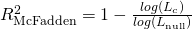where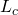denotes the (maximized) likelihood value from the current fitted model, and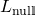denotes the corresponding value but for the null model – the model with only an intercept and no covariates.

To try and understand whether this definition makes sense, suppose first that the covariates in our current model in fact give no predictive information about the outcome. For individual binary data, the likelihood contribution of each observation is between 0 and 1 (a probability), and so the log likelihood contribution is negative. If the model has no predictive ability, although the likelihood value for the current model will be (it is always) larger than the likelihood of the null model, it will not be much greater. Therefore the ratio of the two log-likelihoods will be close to 1, and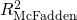will be close to zero, as we would hope.

Next, suppose our current model explains virtually all of the variation in the outcome, which we’ll denote Y. How would this happen? Remembering that the logistic regression model’s purpose is to give a prediction for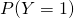for each subject, we would need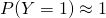for those subjects who did have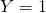, and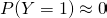for those subjects who had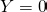. If this is the case, the probability of seeingwhenis almost 1, and similarly the probability of seeingwhenis almost 1. This means that the likelihood value for each observation is close to 1. The log of 1 is 0, and so the log-likelihood value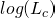will be close to 0. Thenwill be close to 1.

Of course in most empirical research typically one could not hope to find predictors which are strong enough to give predicted probabilities so close to 0 or 1, and so one shouldn’t be surprised if one obtains a value ofwhich is not very large.

## Deterministic or inherently random?

The definition ofalso raises (I think) an interesting philosophical point. From one perspective, we might think of nature (or whatever it is we’re investigating and trying to predict) as deterministic. In this case, our stochastic probability models are models which include randomness which is caused by our imperfect knowledge of predictors or our inability to correctly model their effects on the outcome. From this perspective, the definition ofseems quite appropriate – the gold standard value of 1 corresponds to a situation where we can predict whether a given subject will have Y=0 or Y=1 with almost 100% certainty.

An alternative perspective says that there is, at some level, intrinsic randomness in nature – parts of quantum mechanics theory state (I am told!) that at some level there is intrinsic randomness. Because of this, it will never be possible to predict with almost 100% certainty whether a new subject will have Y=0 or Y=1. In this case, a value of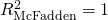will never be attainable. Of course the intrinsic randomness might have a relatively small impact in terms of variability in our outcome.

## McFadden’s R squared in R

In R, the glm (generalized linear model) command is the standard command for fitting logistic regression. As far as I am aware, the fitted glm object doesn’t directly give you any of the pseudo R squared values, but McFadden’s measure can be readily calculated. To do so, we first fit our model of interest, and then the null model which contains only an intercept. We can then calculate McFadden’s R squared using the fitted model log likelihood values:

mod <- glm(y~x, family="binomial")
nullmod <- glm(y~1, family="binomial")
1-logLik(mod)/logLik(nullmod)

Thanks to Brian Stucky for pointing out that the code used in the original version of this article only works for individual binary data.

To get a sense of how strong a predictor one needs to get a certain value of McFadden’s R squared, we’ll simulate data with a single binary predictor, X, with P(X=1)=0.5. Then we’ll specify values for P(Y=1|X=0) and P(Y=1|X=1). Bigger differences between these two values corresponds to X having a stronger effect on Y. We’ll first try P(Y=1|X=0)=0.3 and P(Y=1|X=1)=0.7:

set.seed(63126)
n <- 10000
x <- 1*(runif(n)<0.5)
pr <- (x==1)*0.7+(x==0)*0.3
y <- 1*(runif(n) < pr)
mod <- glm(y~x, family="binomial")
nullmod <- glm(y~1, family="binomial")
1-logLik(mod)/logLik(nullmod)
'log Lik.' 0.1320256 (df=2)

(The value printed is McFadden’s log likelihood, and not a log likelihood!) So, even with X affecting the probability of Y=1 reasonably strongly, McFadden’s R2 is only 0.13. To increase it, we must make P(Y=1|X=0) and P(Y=1|X=1) more different:

set.seed(63126)
n <- 10000
x <- 1*(runif(n)<0.5)
pr <- (x==1)*0.9+(x==0)*0.1
y <- 1*(runif(n) < pr)
mod <- glm(y~x, family="binomial")
nullmod <- glm(y~1, family="binomial")
1-logLik(mod)/logLik(nullmod)
 0.5539419

Even with X changing P(Y=1) from 0.1 to 0.9, McFadden’s R squared is only 0.55. Lastly we’ll try values of 0.01 and 0.99 – what I would call a very strong effect!

set.seed(63126)
n <- 10000
x <- 1*(runif(n)<0.5)
pr <- (x==1)*0.99+(x==0)*0.01
y <- 1*(runif(n) < pr)
mod <- glm(y~x, family="binomial")
nullmod <- glm(y~1, family="binomial")
1-logLik(mod)/logLik(nullmod)
 0.9293177

Now we have a value much closer to 1. Although just a series of simple simulations, the conclusion I draw is that one should really not be surprised if, from a fitted logistic regression McFadden’s R2 is not particularly large – we need extremely strong predictors in order for it to get close to 1. I personally don’t interpret this as a problem – it is merely illustrating that in practice it is difficult to predict a binary event with near certainty.

## Grouped binomial data vs individual data

Sometimes binary data are stored in grouped binomial form. That is, each row in the data frame contains outcome data for a binomial random variable with n>1. This grouped binomial format can be used even when the data arise from single units when groups of units/individuals share the same values of the covariates (so called covariate patterns). For example, if the only covariates collected in a study on people are gender and age category, the data can be stored in grouped form, with groups defined by the combinations of gender and age category. As is well known, one can fit a logistic regression model to such grouped data and obtain the same estimates and inferences as one would get if instead the data were expanded to individual binary data. To illustrate, we first simulate a grouped binomial data frame in R:

data <- data.frame(s=c(700,300),f=c(300,700),x=c(0,1))
data
s   f x
1 700 300 0
2 300 700 1


The simulated data are very simple, with a single covariate x which takes values 0 or 1. The column s records how many ‘successes’ there are and the column ‘f’ records how many failures. There are 1,000 units in each of the two groups defined by the value of the variable x. To fit a logistic regression model to the data in R we can pass to the glm function a response which is a matix where the first column is the number of successes and the second column is the number of failures:

mod1 <- glm(cbind(s,f)~x, family="binomial",data)
summary(mod1)

Call:
glm(formula = cbind(s, f) ~ x, family = "binomial", data = data)

Deviance Residuals:
  0  0

Coefficients:
Estimate Std. Error z value Pr(>|z|)
(Intercept)  0.84730    0.06901   12.28   <2e-16 ***
x           -1.69460    0.09759  -17.36   <2e-16 ***
---
Signif. codes:  0 ‘***’ 0.001 ‘**’ 0.01 ‘*’ 0.05 ‘.’ 0.1 ‘ ’ 1

(Dispersion parameter for binomial family taken to be 1)

Null deviance: 3.2913e+02  on 1  degrees of freedom
Residual deviance: 1.3323e-13  on 0  degrees of freedom
AIC: 18.371

Number of Fisher Scoring iterations: 2


We now convert the grouped binomial data to individual binary (Bernoulli) data, and fit the same logistic regression model. Rather than expanding the grouped data to the much larger individual data frame, we can instead create, separately for x=0 and x=1, two rows corresponding to y=0 and y=1, and create a variable recording the frequency. The frequency is then passed as a weight to the glm function:

individualData <- rbind(cbind(data,y=0),cbind(data,y=1))
individualData$freq <- individualData$s
individualData$freq[individualData$y==0] <- individualData$f[individualData$y==0]
mod2 <- glm(y~x, family="binomial",data=individualData,weight=freq)
summary(mod2)

Call:
glm(formula = y ~ x, family = "binomial", data = individualData,
weights = freq)

Deviance Residuals:
1       2       3       4
-26.88  -22.35   22.35   26.88

Coefficients:
Estimate Std. Error z value Pr(>|z|)
(Intercept)  0.84730    0.06901   12.28   <2e-16 ***
x           -1.69460    0.09759  -17.36   <2e-16 ***
---
Signif. codes:  0 ‘***’ 0.001 ‘**’ 0.01 ‘*’ 0.05 ‘.’ 0.1 ‘ ’ 1

(Dispersion parameter for binomial family taken to be 1)

Null deviance: 2772.6  on 3  degrees of freedom
Residual deviance: 2443.5  on 2  degrees of freedom
AIC: 2447.5

Number of Fisher Scoring iterations: 4


As expected, we obtain the same parameter estimates and inferences as from the grouped data frame. This is because the log likelihood functions are, up to a constant not involving the model parameters, identical. We might expect therefore that McFadden’s R squared would be the same from the two. To calculate this we fit the null models to the grouped data and then the individual data:

nullmod1 <- glm(cbind(s,f)~1, family="binomial",data)
nullmod2 <- glm(y~1, family="binomial",data=individualData,weight=freq)
1-logLik(mod1)/logLik(nullmod1)
'log Lik.' 0.9581627 (df=2)
1-logLik(mod2)/logLik(nullmod2)
'log Lik.' 0.1187091 (df=2)


We see that the R squared from the grouped data model is 0.96, while the R squared from the individual data model is only 0.12. The explanation for the large difference is (I believe) that for the grouped binomial data setup, the model can accurately predict the number of successes in a binomial observation with n=1,000 with good accuracy. In contrast, for the individual binary data model, the observed outcomes are 0 or 1, while the predicted outcomes are 0.7 and 0.3 for x=0 and x=1 groups. The low R squared for the individual binary data model reflects the fact that the covariate x does not enable accurate prediction of the individual binary outcomes. In contrast, x can give a good prediction for the number of successes in a large group of individuals.

An article describing the same contrast as above but comparing logistic regression with individual binary data and Poisson models for the event rate can be found here at the Journal of Clinical Epidemiology (my thanks to Brian Stucky based at the University of Colorado for very useful discussion on the above, and for pointing me to this paper).

In their most recent edition of ’Applied Logistic Regression’, Hosmer, Lemeshow and Sturdivant give quite a detailed coverage of different R squared measures for logistic regression.

### 8 thoughts on “R squared in logistic regression”

1.1) I have fitted an ordinal logistic regression (with only 1 nominal independent variable and 1 response variable). I am not a big fan of the pseudo R2. Anyways, if I want to interpret the Nagelkerke pseudo R2 (=0.066), I can say that the nominal variable explain alone 6.6% of the total (100%) variability of the response variable (=ordinal variable). When I write, at the end of my sentence “variability of the response variable”, I wonder about the word “variability”. Is “variability” referring to the fact that the response variable can vary, in my case, between 3 levels : not important, important and very important ? If not, how could we explain/interpret this sentence “variability of the response variable” ?

2) Regarding the best pseudo R2 value to use, which one would you recommend ? I would recommend Nagelkerke’s index over Cox & Snell’s, as the rescaling results in proper lower and upper bounds (0 and 1). McFadden’s is also a sound index, and very intuitive. However, values of McFadden will typically be lower than Nagelkerke’s for a given data set (and both will be lower than OLS R2 values), so Nagelkerke’s index will be more in line with what most researchers are accustomed to seeing with OLS R2. There is, however, little theoretical or substantive reason other than this for preferring Nagelkerk’s index over McFadden’s, as both perform similarly across varied multicollinearity conditions. Nagelkere’s index, however, might be somewhat more stable at low base rate conditions. Do you agree ?

1) For linear regression, R2 is defined in terms of amount of variance explained. As I understand it, Nagelkerke’s psuedo R2, is an adaption of Cox and Snell’s R2. The latter is defined (in terms of the likelihood function) so that it matches R2 in the case of linear regression, with the idea being that it can be generalized to other types of model. However, once it comes to say logistic regression, as far I know Cox & Snell, and Nagelkerke’s R2 (and indeed McFadden’s) are no longer proportions of explained variance. Nonetheless, I think one could still describe them as proportions of explained variation in the response, since if the model were able to perfectly predict the outcome (i.e. explain variation in the outcome between individuals), then Nagelkerke’s R2 value would be 1.

2) To be honest I don’t know if I’d recommend one over the other – as you say they have different properties and I’m not sure it’s possible to say one is ‘better’ than all the others. In an excellent blog post (http://statisticalhorizons.com/r2logistic), Paul Allison explains why he now doesn’t like Nagelkerke’s adjustment to Cox and Snell because it’s ad-hoc.He then describes yet another recently proposed alternative. Personally, I use McFadden’s R2 as it’s reported in Stata, but I would generally just use it as a rough indicator of how well I am predicting the outcome.

•Thank you for your elaborate expression. Although I am yet a beginner in this area, In have still difficulty even to understand the basic concept and idea of the what of odds, odds ratio, log of odds ratio and the different measures of goodness of fit of a logistic model. Could you present me the meaning of these terms in a simpler language, please?

2.Dear Jonathan,

I really thank you lots for your response. One last precision.
In a multiple linear regression we can get a negative R^2. Indeed, if the chosen model fits worse than a horizontal line (null hypothesis), then R^2 is negative. Now, I have fitted an ordinal logistic regression. I get the Nagelkerke pseudo R^2 =0.066 (6.6%). I use the validate() function from the rms R package to obtain the population corrected index (calculated by bootstrap) and I get a negative pseudo R^2 =-0.0473 (-4.73%). How can we explain that the pseudo R^2 can be negative ?

•In linear regression, the standard R^2 cannot be negative. The adjusted R^2 can however be negative.

If the validate function does what I think (use bootstrapping to estimate the optimism), then I guess it is just taking the naive Nagelkerke R^2 and then subtracting off the estimated optimism, which I suppose has no guarantee of necessarily being non-negative. The interpretation I suppose then for your negative value is that your model doesn’t appear to have much (any) predictive ability.

3.Does McFadden’s pseudo-R2 scale? As in, is a model with R2 = 0.25 2.5x as good as a model with R2 = 0.10?

4.Hi there 🙂
Thank you for these elaborate responses. For my model, Stata gave me a McFadden value of 0.03.

There are few information online as to how to interpret McFadden values with one of the few recommendations being that 0.2 – 0.4 ‘would be excellent’. Other sources say to be weary of pseudo R-square results and not to mistake small values for bad model fit. I am confused about 1. What the value 0.03 tells me
2. If this value is so bad that I should revise my model and/or 3. Despite this low value, am I still able to interprete the coefficients?

5.